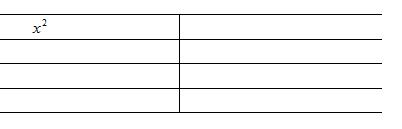# Quod Erat Demonstrandum

## 2012/02/29

### 「新」高中數學練習卷成績

Filed under: NSS — johnmayhk @ 11:43 上午

2012-02-27,28 考評局公佈有關 HKDSE 數學科練習卷（practice papers）學生表現及有關分數的統計數據。聞說考評局從不同 banding 的學校蒐集了差不多 3000 學生的數學必修卷（Core Mathematics）成績及差不多 100 個學生的數學科延伸部分（Module 1 and Module 2）之成績，結果如下：

 Full mark Mean s.d. Sample size Core Math (I) 105 44.8 24.3 3000 Core Math (II) 45 23.3 7.9 3000 M1 100 47.6 19.3 100 M2 100 52.1 20.4 100

## 2012/02/26

### 答網友：y=z^2

Filed under: HKALE,Pure Mathematics — johnmayhk @ 6:29 下午
Tags:

By considering$(1+z)^8+(1-z)^8=0$

show that$\displaystyle\sum_{k=0}^7 \tan^2\frac{(2k+1)\pi}{16}=56$

## 2012/02/25

### 洛必達

Filed under: NSS,Pure Mathematics — johnmayhk @ 5:52 下午

M2 課程沒有 l’Hôpital’s rule，不知是因為課時太緊迫還是學理的原因。$\displaystyle\lim_{x\rightarrow \infty}\frac{x^2+\sin x}{x^2}$$=\displaystyle\lim_{x\rightarrow \infty}(1+\frac{\sin x}{x^2})$$=1$

（因 (more…)

## 2012/02/16

### Core Math 某題：數算 ABC

Filed under: NSS,Teaching — johnmayhk @ 8:58 上午

Select 3 letters from a set {A,A,B,B,B,C,C}. How many different 3-letter ‘words’ can be formed? (e.g. AAB, CBA etc.)$\frac{P^7_3}{2!3!2!}$

## 2012/02/13

### Core Math 某題：概率

Filed under: NSS,Teaching — johnmayhk @ 11:14 上午
Tags:

In a lucky draw of a car, only 1 key out of 10 can open the door of the car. Chris, Rachel and Mike take turns to draw a key at random without replacement. The person who can open the car door will get the car. Find the probabilities of the following events happening.

(a) Chris will get the car.
(b) Rachel will get the car.
(c) Mike will get the car.

## 2012/02/12

### The Foundations of Geometry (David Hilbert)

Filed under: mathematics — johnmayhk @ 10:34 下午

## 2012/02/11

Filed under: Fun — johnmayhk @ 1:07 上午

=_=

## 2012/02/10

### 利用向量證 sine and cosine laws

Filed under: NSS — johnmayhk @ 5:49 下午

1. cosine law$a^2=b^2+c^2-2bc\cos A$$b^2=c^2+a^2-2ca\cos B$$c^2=a^2+b^2-2ab\cos C$(more…)

## 2012/02/09

### 三角矩陣

Filed under: NSS,Pure Mathematics — johnmayhk @ 2:55 下午

The inverse of the product of two non-singular matricesshould be something like

A.B. (more…)

## 2012/02/08

### 重溫：RSA Animate – Changing Education Paradigms

Filed under: Report — johnmayhk @ 6:20 下午

## 2012/02/07

### Integration by parts

Filed under: NSS,Pure Mathematics,Teaching — johnmayhk @ 9:29 上午$\int u(x)v(x)dx$

（其中$u(x)$ 是多項式，$v(x)$ 是容易計算積分的函數）

Evaluate$\int x^2\cos xdx$.## 2012/02/06

### 畫心

Filed under: Fun — johnmayhk @ 3:51 下午http://benice-equation.blogspot.com/2012/02/making-love.html

## 2012/02/05

### [YouTube] Studio 360: Mathematical Art

Filed under: Fun — johnmayhk @ 5:46 下午

## 2012/02/04

### [FW] Fibonacci Flim-Flam

Filed under: Fun,mathematics — johnmayhk @ 8:04 下午(圖，有點兒誤導吧)

## 2012/02/03

### 140 多年前的試卷

Filed under: Fun — johnmayhk @ 4:01 下午http://graphics8.nytimes.com/packages/pdf/education/harvardexam.pdf